# 3 Consider the ordinary differential equation: ty +3tyy 0. e) (2 points) Find the Wronskian Wly,...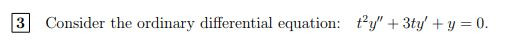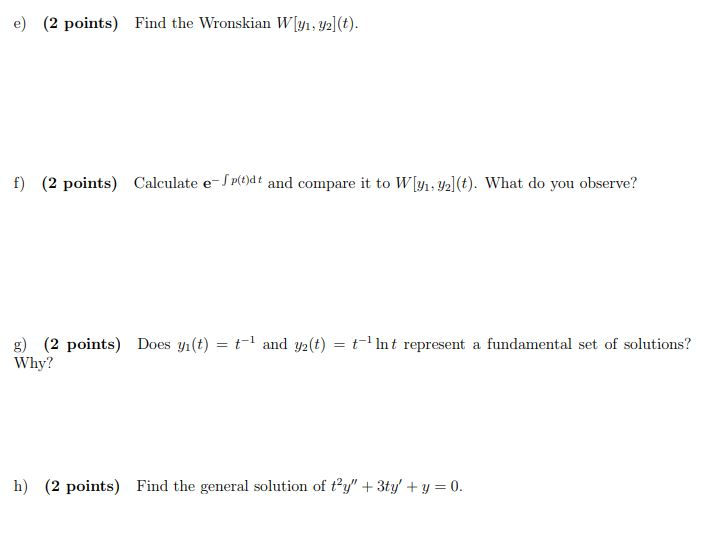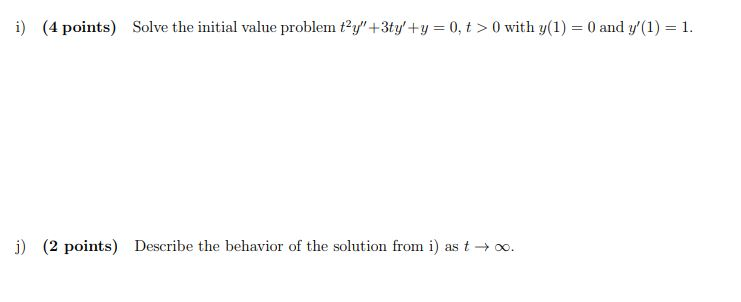3 Consider the ordinary differential equation: ty +3tyy 0.
e) (2 points) Find the Wronskian Wly, yal(t). f) (2 points) Calculate e I podt and compare it to Wl vlt). What do you observe? Does y1(t) = t-1 and y2(t) = t-11nt represent a fundamental set of solutions? g) (2 points) Why? h) (2 points) Find the general solution of ty" +3ty'y 0
İ) (4 points) Solve the initial value problem t2y't3ty'+y = 0, t > 0 with y(1) = 0 and y(1-1. j) (2 points) Describe the behavior of the solution from i) as t 00.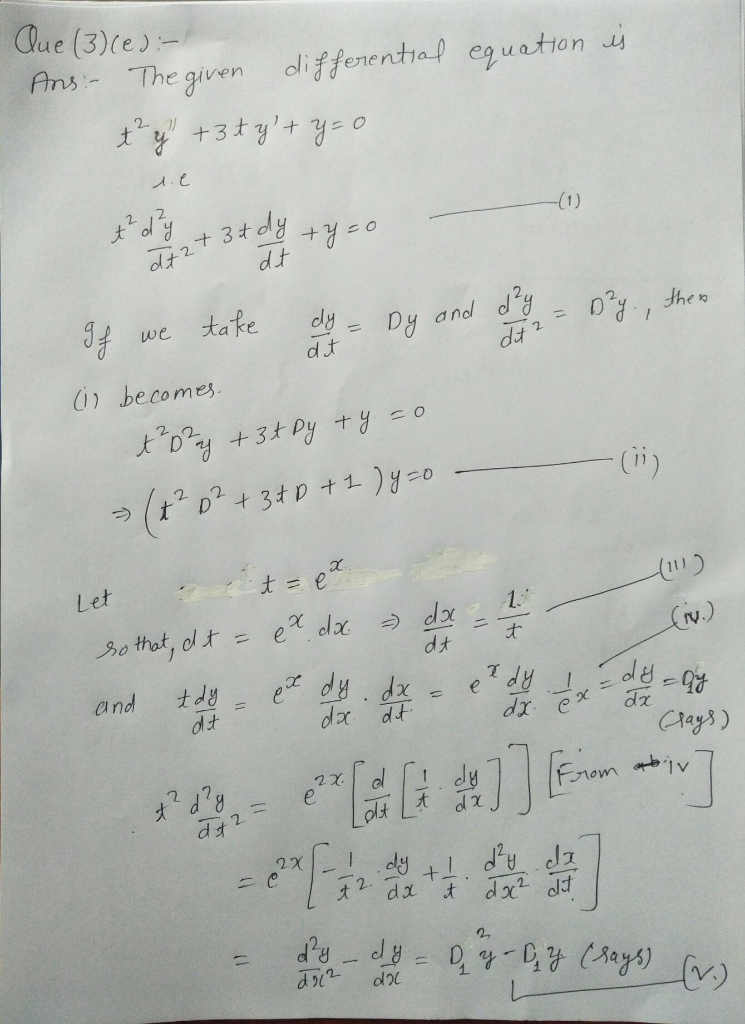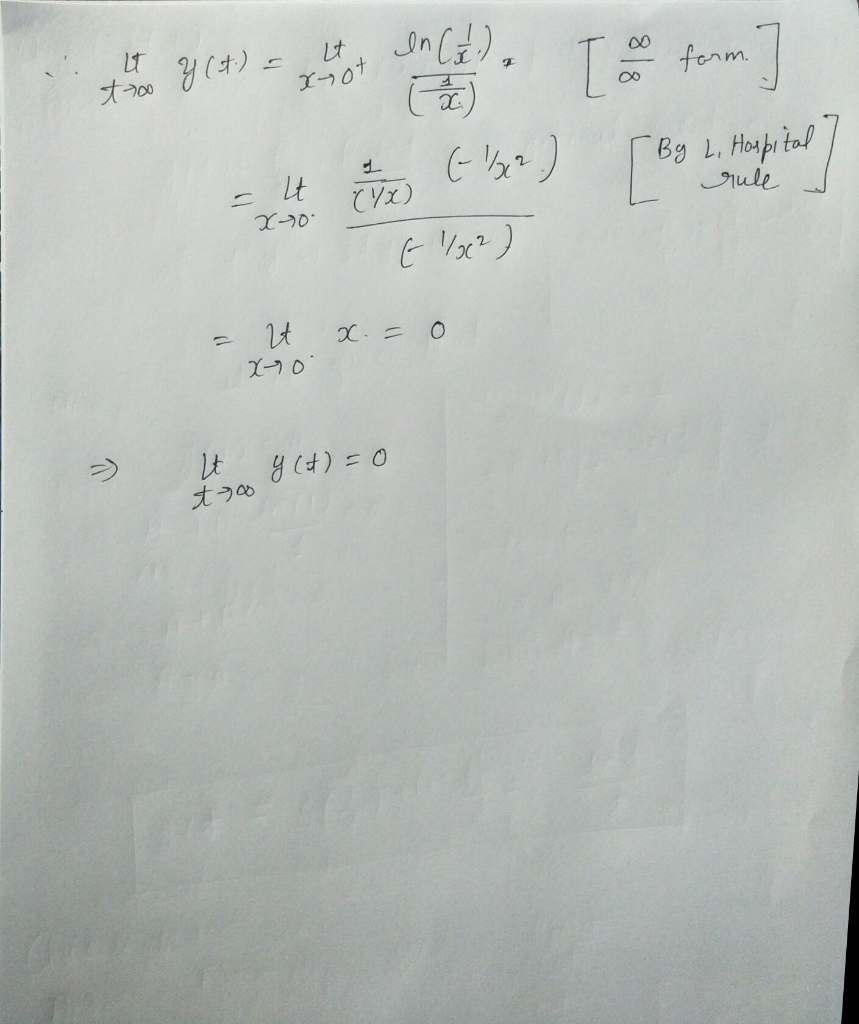#### Earn Coin

Coins can be redeemed for fabulous gifts.

Similar Homework Help Questions
• ### Consider the ordinary differential equation: t2y" + 3ty' +y = 0. 1 (3 points) e) Use...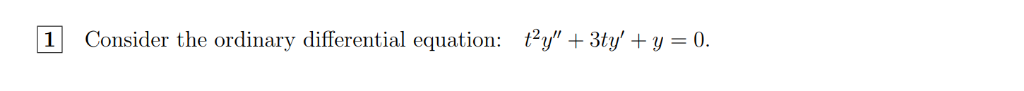Consider the ordinary differential equation: t2y" + 3ty' +y = 0. 1 (3 points) e) Use Abel's formula to find the Wronskian of any two solutions of this equation and W[y1,y2](t). What do you observe? compare it to = t1 and y2(t) = t-1 nt represent a fundamental set of solu f) (2 points) Determine if y1 (t) tions (2 points) Find the general solution of t2y" +3ty' +y = 0. g) Solve the initial value problem t2y" + 3ty/...

• ### Bonus (Abel's formula) a) Show that if y1 and y2 are solutions to the differential equation...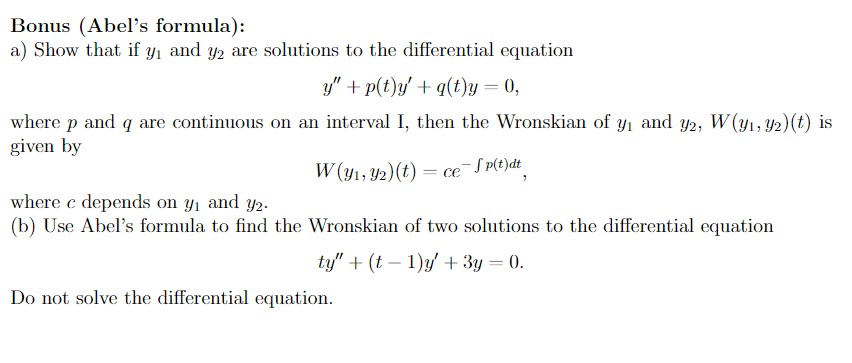Bonus (Abel's formula) a) Show that if y1 and y2 are solutions to the differential equation y"p(t)y(t)y 0 where p and q are continuous on an interval I, then the Wronskian of y and y2, W(y1,y2) (t) is given by - Sp(t)dt ce W(y1, y2)(t) where c depends on y and y2 (b) Use Abel's formula to find the Wronskian of two solutions to the differential equation ty"(t 1)y 3y 0 Do not solve the differential equation

• ### #2 Problem 2 . If the Wronskian of f and g is tcost - sint and...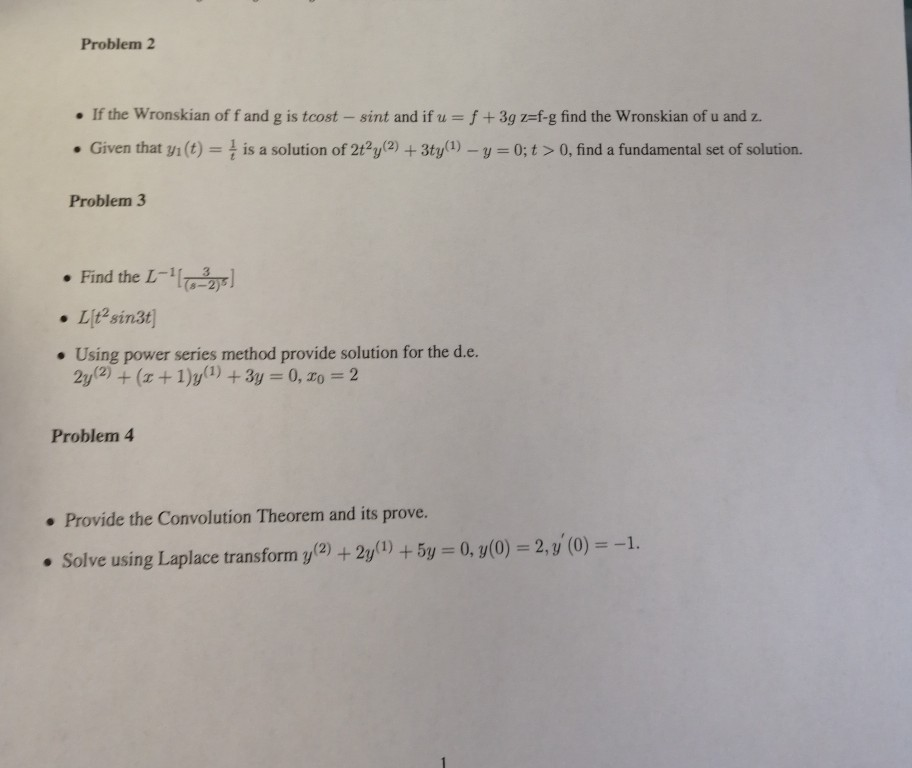#2 Problem 2 . If the Wronskian of f and g is tcost - sint and if u f+3g z-f-g find the Wronskian of u and z. 0, find a fundamental set of . Given that i(t) is a solution of 2t2y(2) +3ty1) -y 0;t> solution. Problem 3 . Find the L-11 . Using power series method provide solution for the d.e. Problem 4 . Provide the Convolution Theorem and its prove. ve using Laplace transform y2 +2y+5y 0, y(0)...

• ### A) Assume that y1(c) t and y2)te are solutions of the differential equation t2y_ t(t + 2))" + t(t...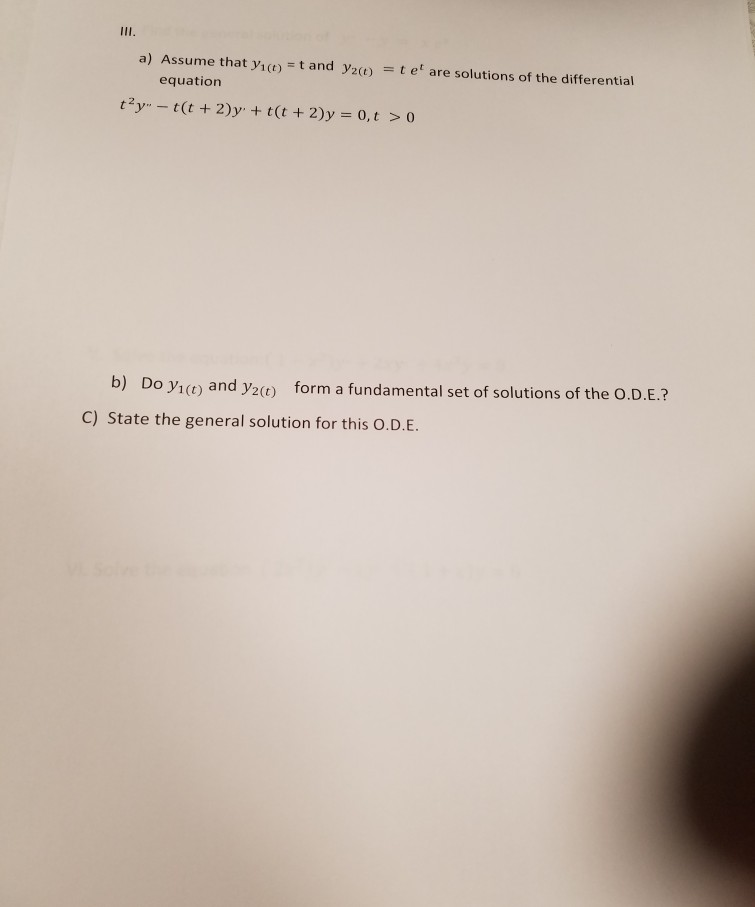a) Assume that y1(c) t and y2)te are solutions of the differential equation t2y_ t(t + 2))" + t(t + 2)y-0, t > 0 Do y1(t) and y2() form a fundamental set of solutions of the O.D.E.? C) State the general solution for this O.D.E. a) Assume that y1(c) t and y2)te are solutions of the differential equation t2y_ t(t + 2))" + t(t + 2)y-0, t > 0 Do y1(t) and y2() form a fundamental set of solutions of...

• ### h Bessel equation of order p is ty" + ty + (t? - p2 y =...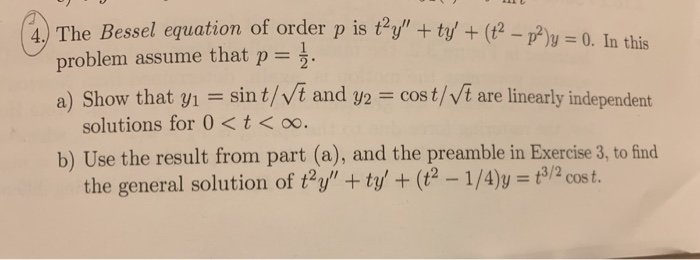h Bessel equation of order p is ty" + ty + (t? - p2 y = 0. In this problem assume that p= 2. a) Show that y1 = sint/Vt and y2 = cost/vt are linearly independent solutions for 0 <t<o. b) Use the result from part (a), and the preamble in Exercise 3, to find the general solution of ty" + ty' + (t2 - 1/4)y = 3/2 cost. (o if 0 <t < 12, y(t) = { 2...

• ### 3. Consider the differential equation ty" - (t+1)y + y = t?e?', t>0. (a) Find a...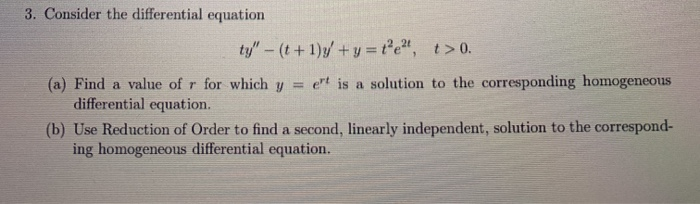3. Consider the differential equation ty" - (t+1)y + y = t?e?', t>0. (a) Find a value ofr for which y = et is a solution to the corresponding homogeneous differential equation. (b) Use Reduction of Order to find a second, linearly independent, solution to the correspond- ing homogeneous differential equation. (c) Use Variation of Parameters to find a particular solution to the nonhomogeneous differ- ential equation and then give the general solution to the differential equation.

• ### Here are the question and answer of ordinary differential equation. Please show the steps. Thanks! 5....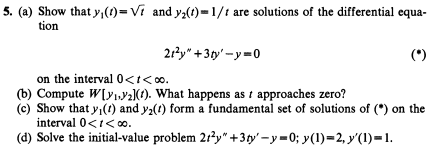Here are the question and answer of ordinary differential equation. Please show the steps. Thanks! 5. (a) Show that yo-Vi and y(t)-1/1 are solutions of the differential equa- tion (*) 21%y+3 ty' - y=0 on the interval 0<t<0. (6) Compute W[ 2](). What happens as i approaches zero? (c) Show that y(i) and yz(1) form a fundamental set of solutions of (*) on the interval 0<t<0. (d) Solve the initial-value problem 21%y" +3 ty'-y 0; y(1)-2, y'(l)-1. 5. (b) W=32;...

• ### dy: 2 Consider the following Ordinary Differential Equation (ODE) for function yı(z) on interval [0, 1]...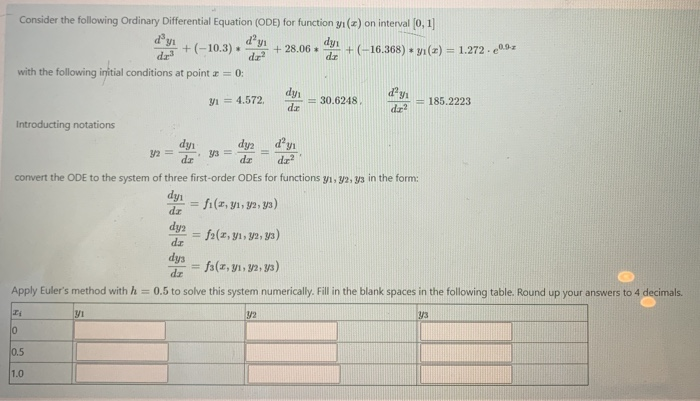dy: 2 Consider the following Ordinary Differential Equation (ODE) for function yı(z) on interval [0, 1] +(-10,3) dayi dy + 28.06 + (-16.368) + y(x) = 1.272.0.52 with the following initial conditions at point a = 0; dy 91 = 4.572 = 30.6248 = 185.2223 dar Introducting notations dyi dy2 dy dar dar dir? convert the ODE to the system of three first-order ODEs for functions y1, y2, y3 in the form: dy dar fi (1, y1, ya, y) dy2...

• ### Consider the differential equation e24 y" – 4y +4y= t> 0. t2 (a) Find T1, T2,...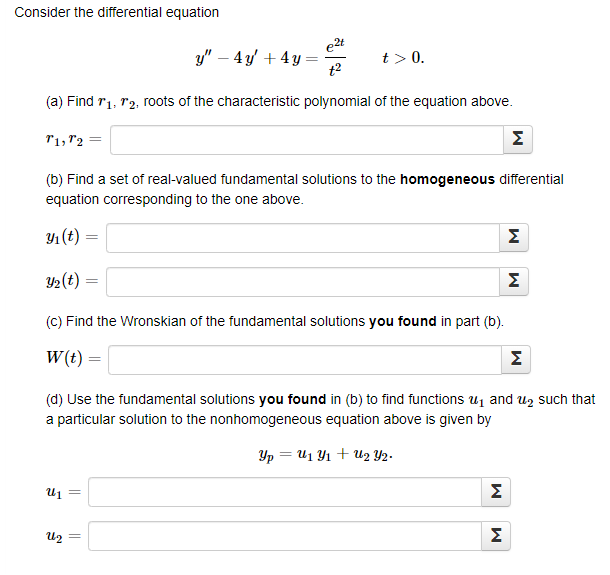Consider the differential equation e24 y" – 4y +4y= t> 0. t2 (a) Find T1, T2, roots of the characteristic polynomial of the equation above. 11,12 M (b) Find a set of real-valued fundamental solutions to the homogeneous differential equation corresponding to the one above. yı(t) M y2(t) = M (C) Find the Wronskian of the fundamental solutions you found in part (b). W(t) M (d) Use the fundamental solutions you found in (b) to find functions ui and Usuch...

• ### Consider the differential equation: -9ty" – 6t(t – 3)y' + 6(t – 3)y=0, t> 0. a....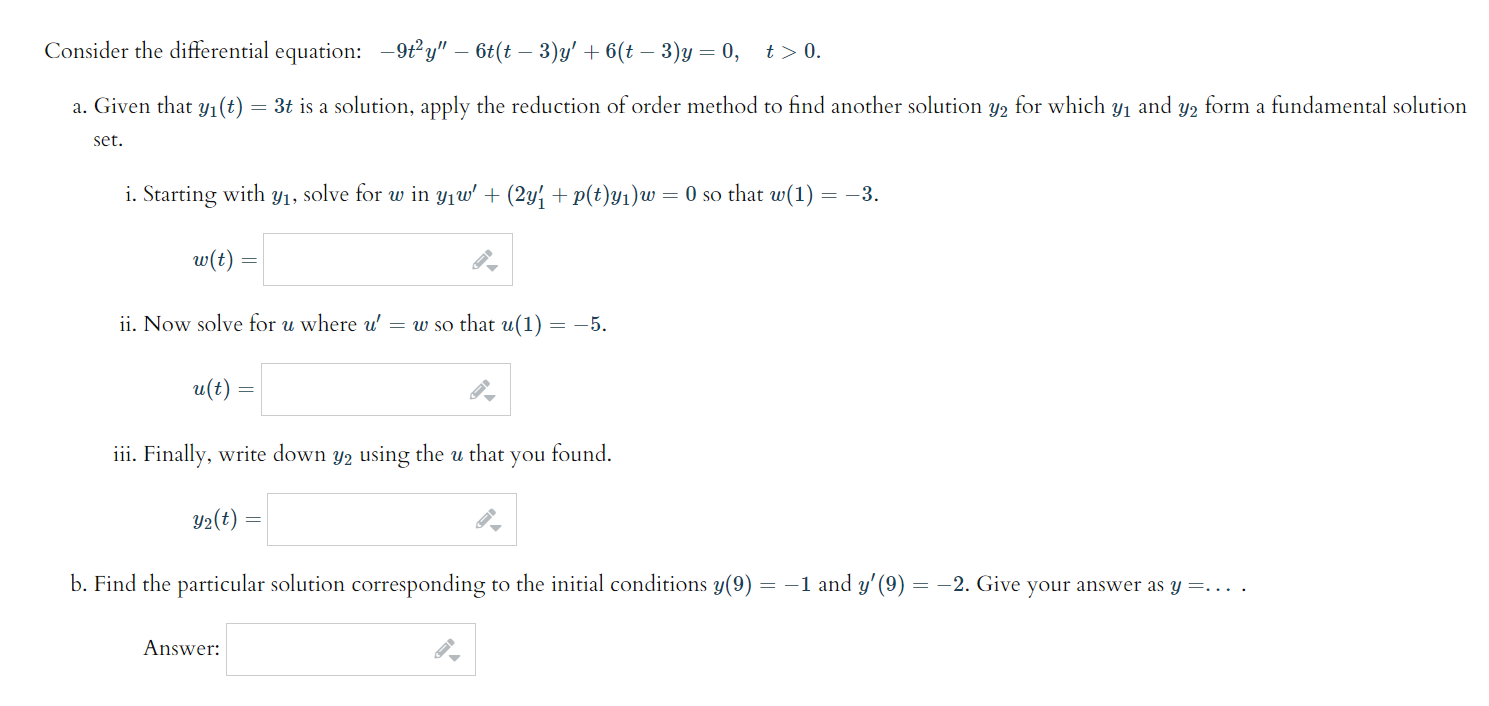Consider the differential equation: -9ty" – 6t(t – 3)y' + 6(t – 3)y=0, t> 0. a. Given that yı(t) = 3t is a solution, apply the reduction of order method to find another solution y2 for which yı and y2 form a fundamental solution set. i. Starting with yi, solve for w in yıw' + (2y + p(t)yı)w = 0 so that w(1) = -3. w(t) = ii. Now solve for u where u = w so that u(1) =...